# What are electric lines of force - Properties of lines of forces.

Hey, do you want to learn about What are electric lines of force? If yes. Then keep reading.

## Electric Lines of Force

The idea of lines of force was introduced by Michel Faraday. A line of force is an imaginary curve the tangent to which at a point gives the direction of intensity at that point and the number of lines of force per unit area normal to the surface surrounding that point gives the magnitude of intensity at that point.

### Important points

1. Electric lines of force usually start or diverge out from a positive charge and end or converge on a negative charge.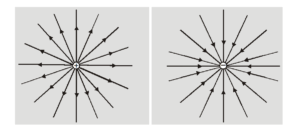2. The number of lines originating or terminating on a charge is proportional to the magnitude of the charge. In SI units $1 / \varepsilon_{0}$ shows electric lines associated with unit (i.e., 1 coulomb) charge So if a body encloses a charge q, total lines of force or flux associated with it is $\mathrm{q} / \varepsilon_{\mathrm{p}}$. If the body is cubical and the charge is situated at its center the lines of force through each face will be $\mathrm{q} / 6 \varepsilon_{0}$.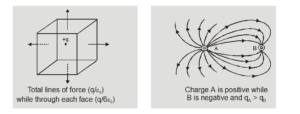3. Lines of force never cross each other because if they cross, then intensity at that point will have two directions which are not possible.
4. In electrostatics, the electric lines of force can never be closed loops, as a line can never start and end on the same charge. If a line of force is a closed curve, work done round a closed path will not be zero and the electric field will not remain conservative.
5. Lines of force have a tendency to contract longitudinally like a stretched elastic string producing attraction between opposite charges and repel each other laterally resulting in, the repulsion between similar charges and 'edge-effects (curving of lines of force near the edges of a charged conductor)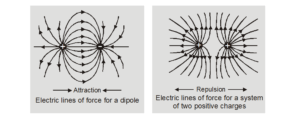6. If the lines of force are equidistant straight lines the field is uniform and if lines of force are not equidistant or straight lines or both, the field will be non-uniform. The first three represent a non-uniform field while the last shows a uniform field.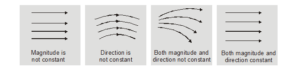7. Electric lines of force end or start normally on the surface of a conductor. If a line of force is not normal to the surface of a conductor, the electric intensity will have a component along the surface of the conductor and hence conductor will not remain equipotential which is not possible as in electrostatics conductor is an equipotential surface.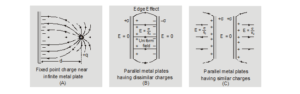8. If in a region of space, there is no electric field there will be no lines of force. This is why inside a conductor or at a neutral point where resultant intensity is zero there is no line of force.
9. The number of lines of force per unit normal area at a point represents the magnitude of electric field intensity. The crowded lines represent a strong field while distant lines show a weak field.
10. The tangent to the line of force at a point in an electric field gives the direction of intensity. It gives the direction of the force and hence acceleration which a positive charge will experience there (and not the direction of motion). A positive point charge-free to move may or may not follow the line of force. It will follow the line of force if it is a straight line (as the direction of velocity and acceleration will be the same) and will not follow the line if it is curved as the direction of motion will be different from that of acceleration. The particle will not move in the direction of motion or acceleration (line of force) but other than these which will vary with time as $\overrightarrow{\mathrm{v}}=\overrightarrow{\mathrm{u}}+\overrightarrow{\mathrm{at}}$

## Comparison of electric and magnetic lines of force

1. Electric lines of force never form closed loops while magnetic lines of force are always closed loops.
2. Electric lines of force always emerge or terminate normally on the surface of a charged conductor, while magnetic lines of force start or terminate on the surface of magnetic material at any angle.
3. Electric lines of force do not exist inside a conductor, but magnetic lines of force may exist inside magnetic material.
4. Total electric lines of force linked with a closed surface may or may not be zero, but total magnetic lines of force linked with a closed surface is always zero (as monopolies do not exist).

So, that's all from this article. I hope you get the idea about What are electric lines of force. If you liked this article then please share it with your friends. If you have any confusion related to this topic then feel free to ask in the comments section down below.

For a better understanding of this chapter, please check the detailed notes of the Electric charge and Fields. To watch Free Learning Videos on physics by Saransh Gupta sir Install the eSaral App.